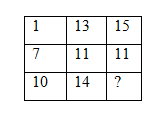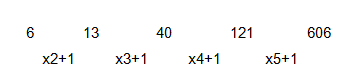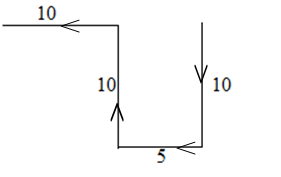# SSC CGL 2018 Practice Test Papers | Reasoning Ability (Day-22)

Dear Aspirants, Here we have given the Important SSC CGL Exam 2018 Practice Test Papers. Candidates those who are preparing for SSC CGL 2018 can practice these questions to get more confidence to Crack SSC CGL 2018 Examination.

[WpProQuiz 2997]

Click “Start Quiz” to attend these Questions and view Explanation

1. Select the related letters from the given alternatives.

BDF: KMO:: SUW:?

a) ACE

b) WAY

c) BDF

d) None of these

2.  Find the odd one

a) 63679

b) 86394

c) 57387

d) 71819

3. Select the related letters from the given alternatives.

1:8 :: ?? :64

a) 49

b) 81

c) 5

d) 3

4. A series is given, with one term missing. Choose the correct alternative from the given ones that will complete the series

a) 560

b) 309

c) 606

d) 720

5. Some equations are solved on the basis of certain system. Find out the correct answer for the unsolved equation on that basis.
If, 3*2*3*4=14, 4*5*1*10=10 then 6*3*2*15=?

a) 19

b) 23

c) 45

d) 24

6. From the given alternative words, select the word which cannot be formed using the letters of the given word:

MARVELOUS

a) RAM

b) MARE

c) FAMOUS

d) SUM

7. Arrange the following words as per order in the dictionary

i) Igloo

ii) Infrared

iii) Island

iv) Ireland

a) i), ii), iv), iii)

b) ii), iii), iv), ii)

c) i), ii), iii), iv)

d) iv), i), ii), iii)

8. If SNOW is written as QRLB, then how SOFA may be written in that code?

a) TUOD

b) QSCF

c) LMGT

d) RAGU

9. Aswin walked 10 km south and turned right and walked 5 km. Then again he turned right and walked 10 km. Then he turned left and walked 10 km. How many km will Aswin have to walk to reach the starting point?

a) 20

b) 15

c) 25

d) 5

10. Select the missing number in the following table.a) 29

b) 5

c) 13

d) 20

B+2=D, D+2=F, F+5=K, K+2=M, M+2=O

S+2=U, U+2=W, W+5=B, B+2=D, D+2=F

All numbers except D are multiples of 11.

1:8  =>1+1=2, 2^3=8

3:64=>3+1=4, 4^3=643 x 2 x 3 – 4 =14

4 x 5 x 1 – 10 = 10

6 x 3 x 2 – 15 = 19

FAMOUS cannot be formed since F is not present in the given word.

i) Igloo

ii) Infrared

iv) Ireland

iii) Island

S – 2 = Q

N + 4 = R

O – 3 = L

W + 5 = B

And

S – 2 = Q

O + 4 = S

F – 3 = C

A + 5 = F